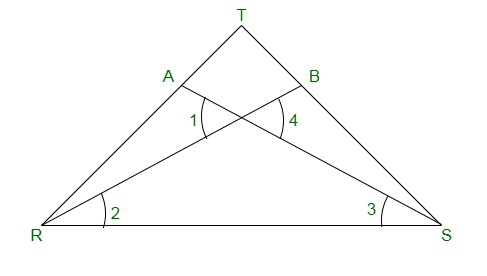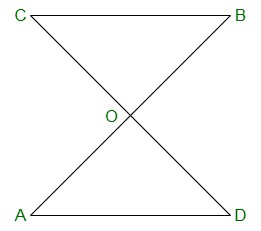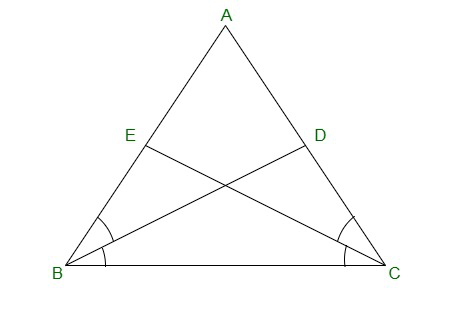# Class 9 RD Sharma Solutions – Chapter 10 Congruent Triangles- Exercise 10.2

### Question 1: In figure, it is given that RT = TS, ∠1 = 2∠2 and ∠4 = 2(∠3). Prove that ΔRBT ≅ ΔSAT.

Solution:

In the figure,
Given:
RT = TS —-> (equation 1)
∠1 = 2 ∠2 —-> (equation 2)
∠4 = 2 ∠3 —->(equation 3)To prove:
ΔRBT ≅ ΔSAT

Let the point of intersection RB and SA be denoted by O
∠AOR = ∠BOS [Vertically opposite angles]
So, ∠1 = ∠4
2 ∠2 = 2 ∠3 [From (equation 2) and (equation 3)]
or
∠2 = ∠3 —->(equation 4)

Now in Δ TRS,
We have RT = TS
Δ TRS is an isosceles triangle
∠TRS = ∠TSR —->(equation 5)
But, ∠TRS = ∠TRB + ∠2 —->(equation 6)
∠TSR = ∠TSA + ∠3 —->(equation 7)
Putting (equation 6) and (equation 7) in (equation 5) we get
∠TRB + ∠2 = ∠TSA + ∠3
∠TRB = ∠TSA [From (equation 6)]
Consider ΔRBT and ΔSAT
RT = ST [From (equation 1)]
∠TRB = ∠TSA [From (equation 6)]
By ASA criterion of congruence, we have
ΔRBT ≅ ΔSAT

### Question 2: Two lines AB and CD intersect at O such that BC is equal and parallel to AD. Prove that the lines AB and CD bisect at O.

Solution:

Given:
Lines AB and CD Intersect at O
Such that BC ∥ AD andTo prove:
AB and CD bisect at O.

First we have to prove that ΔAOD ≅ ΔBOC
∠OCB =∠ODA {AD||BC and CD is transversal}
AD = BC {from (equation 1)}
By ASA Criterion:
ΔAOD ≅ ΔBOC
OA = OB and OD = OC (By Corresponding parts of congruent triangle )
Therefore,
AB and CD bisect each other at O.
Hence, Proved.

### Question 3: BD and CE are bisectors of ∠B and ∠C of an isosceles Δ ABC with AB = AC. Prove that BD = CE.

Solution:

Given:
ΔABC is isosceles
Where,
AB = AC
BD and CE are bisectors of ∠ B and ∠ CTo prove:
BD = CE

Since AB = AC (given)
∠ABC = ∠ACB —->(equation 1) {Angles opposite to equal sides are equal}
Since BD and CE are bisectors of ∠ B and ∠ C
∠ABD = ∠DBC =∠ BCE =ECA =  ∠B  = ∠C   —-> (equation 2)
2        2
Now, Consider ΔEBC = ΔDCB
∠EBC = ∠DCB {From (equation 1)}
BC = BC {Common side}
∠BCE = ∠CBD {From (equation 2)}
By ASA congruence criterion,
Δ EBC ≅ Δ DCB
Since corresponding parts of congruent triangles are equal.(c.p.c.t.)
CE = BD
or,
BD = CE
Hence, proved.

Whether you're preparing for your first job interview or aiming to upskill in this ever-evolving tech landscape, GeeksforGeeks Courses are your key to success. We provide top-quality content at affordable prices, all geared towards accelerating your growth in a time-bound manner. Join the millions we've already empowered, and we're here to do the same for you. Don't miss out - check it out now!

Previous
Next